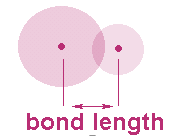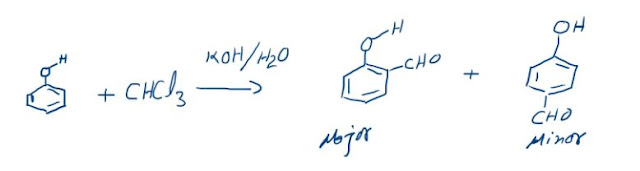## Properties of Covalent Bond

### Bond Length:

Internuclear distance between two atoms in a molecule.
Bond length in covalent compound (AB) = rA + rB### FACTORS AFFECTING BOND LENGTH

I. Atomic size:
Bond length α Atomic size
HF < HCl < HBr < HI: Bond length
F2 < Cl2 < Br2 < I2

2. The lengths of double bonds are less than the lengths of single bonds between the same two atoms, and triple bonds are even shorter than double bonds.
Single bond > Double bond > Triple bond
(Decreasing bond length)

3. Bond length decreases with increase in s-character since s-orbital is smaller than a p – orbital.
sp3 C – H = 1.112 Å    sp2 C – H = 1.103 Å     sp C – H = 1.08 Å

### Bond energy or bond strength:

Bond energy or bond strength is defined as the amount of energy required to break a bond in molecule.
Important features of bond energy:
·       The magnitude of the bond energy depends on the type of bonding. Most of the covalent bonds have energy between 50 to 100 kcal mol–1 (200-400 kJ mol–1). Strength of sigma bond is more than that of a π-bond.
·       A double bond in a diatomic molecules has a higher bond energy than a single bond and a triple bond has a higher bond energy than a double bond between the same atoms. C ≡ C > C = C > C – C (decreasing bond length)
·       The magnitude of the bond energy depends on the size of the atoms forming the bond, i.e. bond length. Shorter the bond length, higher is the bond energy.

·       Bond energy decreases down the group in case of similar molecules.

·       Bond energy increase in the following order:

s < p < sp < sp2 < sp3
C – C          >         N – N         >          O – O
(No lone pair)        (One lone pair)              (Two lone pair)

### Bond Angles

Angle between two adjacent bonds at an atom in a molecule made up of three or more atoms is known as the bond angle.
Bond angles mainly depend on the following three factors:

(i) Hybridization: Bond angle depends on the state of hybridization of the central atom
Generally as the s- character increase in the hybrid bond, the bond angle also increases.
Hybridization         Bond Angle
SP                          180o
SP2                                    120o
SP3                                     109.5 o

(ii) Lone pair repulsion: Bond angle is affected by the presence of lone pair of electrons at the central atom. A lone pair of electrons at the central atom always tries to repel the shared pair (bonded pair) of electrons. Due to this, the bonds are displaced slightly inside resulting in a decrease of bond angle.

(iii) Electronegativity: If the electronegativity of the central atom decreases, bond angle decreases.

### The Reimer-Tiemann reaction: Reaction and its Mechanism

Reimer-Tiemann Reaction Phenols on reaction with chloroform in the presence of sodium hydroxide (or potassium hydroxide) solution give hydro...